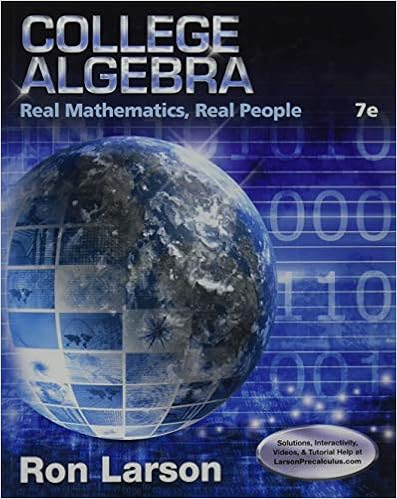hw1 - Math 10C Homework 1 due Fri Jan 11 Please complete...

• Notes
• 2

This preview shows page 1 - 2 out of 2 pages.

We have textbook solutions for you!
The document you are viewing contains questions related to this textbook.The document you are viewing contains questions related to this textbook.
Chapter P / Exercise 048
College Algebra: Real Mathematics, Real People
LarsonExpert Verified
Math 10C Homework 1 due Fri Jan 11 Please complete the problem listed from your textbook and the exercises below. Section 13.1: 2, 4, 6, 7, 12, 16, 25, 30 1. The following is a picture of a box that has the origin as a corner and as the opposite corner. The top side of the box is contained in the plane (a) Find the equation of the plane containing the left side of the box. (b) Find the equation of the plane containing the right side of the box. (c) Find the equation of the plane containing the back side of the box. (d) Find the equation of the plane containing the front side of the box. 2. Consider the points (a) Are and points in or in ? (b) Find .
We have textbook solutions for you!
The document you are viewing contains questions related to this textbook.The document you are viewing contains questions related to this textbook.
Chapter P / Exercise 048
College Algebra: Real Mathematics, Real People
LarsonExpert Verified
(c) Draw as a located vector. That is, draw as a vector beginning at and ending at . (d) Find . (e) Draw as a located vector. (f) Find .
•••